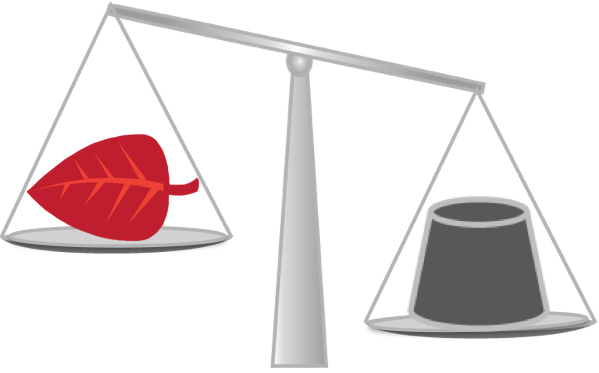# How to Find Volumetric Mass Density (Metric)

This entry uses the metric system. For the imperial system click here.

Mass density is a topic you may recognize from science class. The mass density of a substance says something about how compact a substance is. This means that you can have two objects that are the same size (volume), but due to different densities, one object will weigh more (have larger mass) than the other. Mass density is given by mass ($m$) divided by volume ($V$). The symbol for mass density is the Greek letter $\rho$ (rho).

Rule

### MassDensity:MassperVolume

 $\rho =\frac{m}{V}$Example 1

Iron has a mass density of $\rho =\text{}7.88\text{}\phantom{\rule{0.17em}{0ex}}{\text{g/cm}}^{3}$. An iron cannon ball has a volume of $\text{}1800\text{}\phantom{\rule{0.17em}{0ex}}{\text{cm}}^{3}$. What does the cannon ball weigh?

Insert the values into the formula and solve for $m$: $\begin{array}{llll}\hfill \rho =\frac{m}{V}⇔m& =\rho \cdot V\phantom{\rule{2em}{0ex}}& \hfill & \phantom{\rule{2em}{0ex}}\\ \hfill & =7.88\phantom{\rule{0.17em}{0ex}}{\text{g/cm}}^{3}\cdot 1800\phantom{\rule{0.17em}{0ex}}{\text{cm}}^{3}\phantom{\rule{2em}{0ex}}& \hfill & \phantom{\rule{2em}{0ex}}\\ \hfill & =14\phantom{\rule{0.17em}{0ex}}184\phantom{\rule{0.17em}{0ex}}\text{g}\phantom{\rule{2em}{0ex}}& \hfill & \phantom{\rule{2em}{0ex}}\\ \hfill & \approx 14\phantom{\rule{0.17em}{0ex}}\text{kg}\phantom{\rule{2em}{0ex}}& \hfill & \phantom{\rule{2em}{0ex}}\end{array}$

Mass density is measured in ${\text{kg/m}}^{3}$, but there are also many other units in use. A unit you may have seen before, is ${\text{g/cm}}^{3}$. Water has a mass density equal to $1\phantom{\rule{0.17em}{0ex}}{\text{g/cm}}^{3}$. If an object has a lower mass density than water, it will float in the water. However, if an object has a higher mass density than water, it will sink.

Example 2

A plank weighs $\text{}1500\text{}\phantom{\rule{0.17em}{0ex}}\text{g}$, and has a volume of $\text{}2.75\text{}\phantom{\rule{0.17em}{0ex}}{\text{dm}}^{3}$. Will the plank float in water?

First, make sure the units are the same:

 $2.75\phantom{\rule{0.17em}{0ex}}{\text{dm}}^{3}=\left(2,75\cdot 1{0}^{3}\right)\phantom{\rule{0.33em}{0ex}}{\text{cm}}^{3}=2750\phantom{\rule{0.17em}{0ex}}{\text{cm}}^{3}$

The plank has mass density

 $\rho =\frac{m}{V}=\frac{1500\phantom{\rule{0.17em}{0ex}}\text{g}}{2750\phantom{\rule{0.17em}{0ex}}{\text{cm}}^{3}}\approx 0.55\phantom{\rule{0.17em}{0ex}}{\text{g/cm}}^{3}$

The plank has a much lower mass density than water, which means it will float.

Example 3Annie has found two bars in a hidden cabinet. They are gold colored, and Annie wonders if she has found real gold bars, or if they have been painted on the outside. She has weighed and measured the volume of one of the bars. It weighed $12\phantom{\rule{0.17em}{0ex}}\text{kg}=12\phantom{\rule{0.17em}{0ex}}000\phantom{\rule{0.17em}{0ex}}\text{g}$, and had a volume of about $622\phantom{\rule{0.17em}{0ex}}{\text{cm}}^{3}$. Annie has found that the mass density of gold is $19.3\phantom{\rule{0.17em}{0ex}}{\text{g/cm}}^{3}$. Is it a real gold bar? $\begin{array}{llll}\hfill \rho & =\frac{m}{V}\phantom{\rule{2em}{0ex}}& \hfill & \phantom{\rule{2em}{0ex}}\\ \hfill & =\frac{12\phantom{\rule{0.17em}{0ex}}000\phantom{\rule{0.17em}{0ex}}\text{g}}{622\phantom{\rule{0.17em}{0ex}}{\text{cm}}^{3}}\phantom{\rule{2em}{0ex}}& \hfill & \phantom{\rule{2em}{0ex}}\\ \hfill & \approx 19.29\phantom{\rule{0.17em}{0ex}}{\text{g/cm}}^{3}\phantom{\rule{2em}{0ex}}& \hfill & \phantom{\rule{2em}{0ex}}\\ \hfill & \approx 19.3\phantom{\rule{0.17em}{0ex}}{\text{g/cm}}^{3}\phantom{\rule{2em}{0ex}}& \hfill & \phantom{\rule{2em}{0ex}}\end{array}$

Annie’s bar definitely seems to be made of gold.